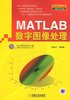matlab数字图像处理 订阅
《MATLAB数字图像处理》是2009年机械工业出版社出版的图书，作者是张德丰。 展开全文《MATLAB数字图像处理》是2009年机械工业出版社出版的图书，作者是张德丰。

ISBN
9787111257356

39.00 元

MATLAB数字图像处理

2009年

16

MATLAB数字图像处理内容简介
《MATLAB数字图像处理》详细介绍了数字图像处理技术及利用MATLAB进行图像处理的方法和技巧，强调了图像处理的理论和应用相结合的方法，并给出了大量数字图像处理技术的MATLAB实现程序。在实例中强调了如何用MATLAB图像处理工具箱解决图像处理中的问题、难题，节省了图像处理的时间，提高了图像处理的效率。《MATLAB数字图像处理》可作为高等理工科院校电子信息、通信工程、信号与信息处理学科的本科生教材，也可作为研究生以及从事图像研究的科研工作者的学习参考用书。...

# matlab数字图像处理matlab 订阅